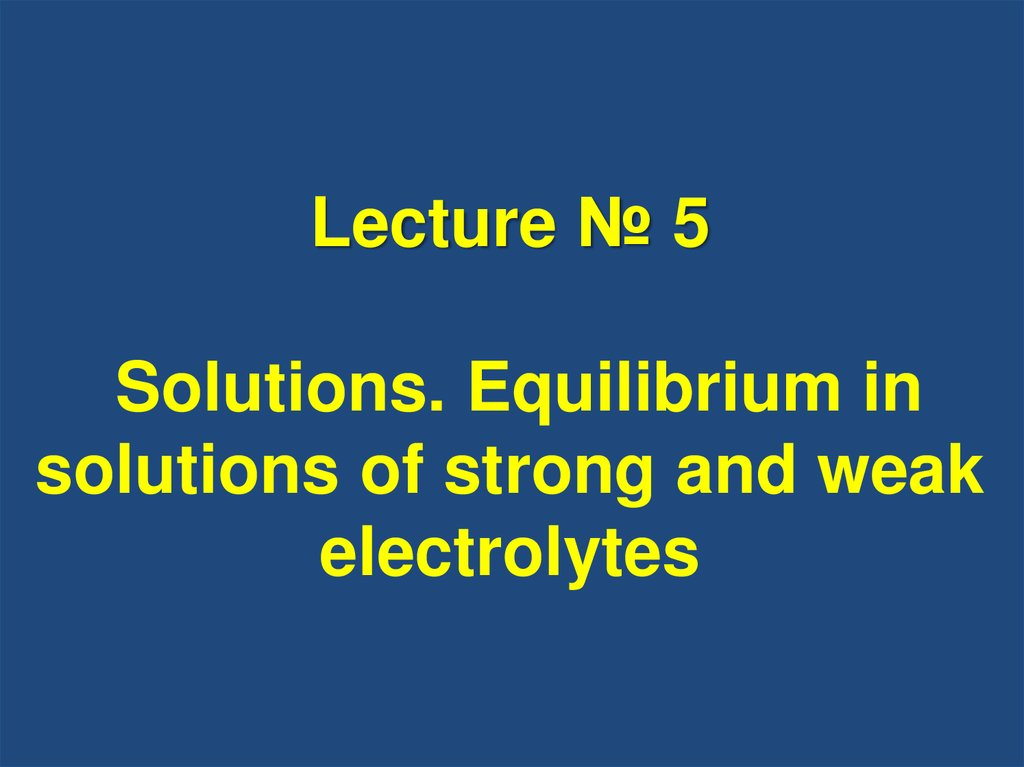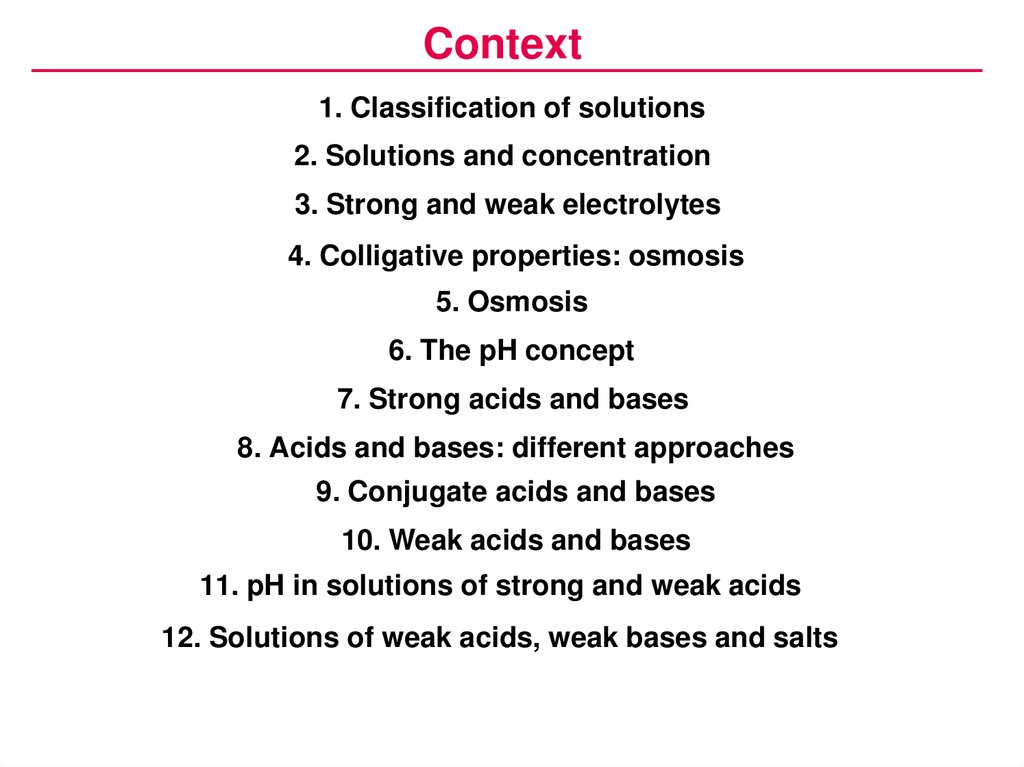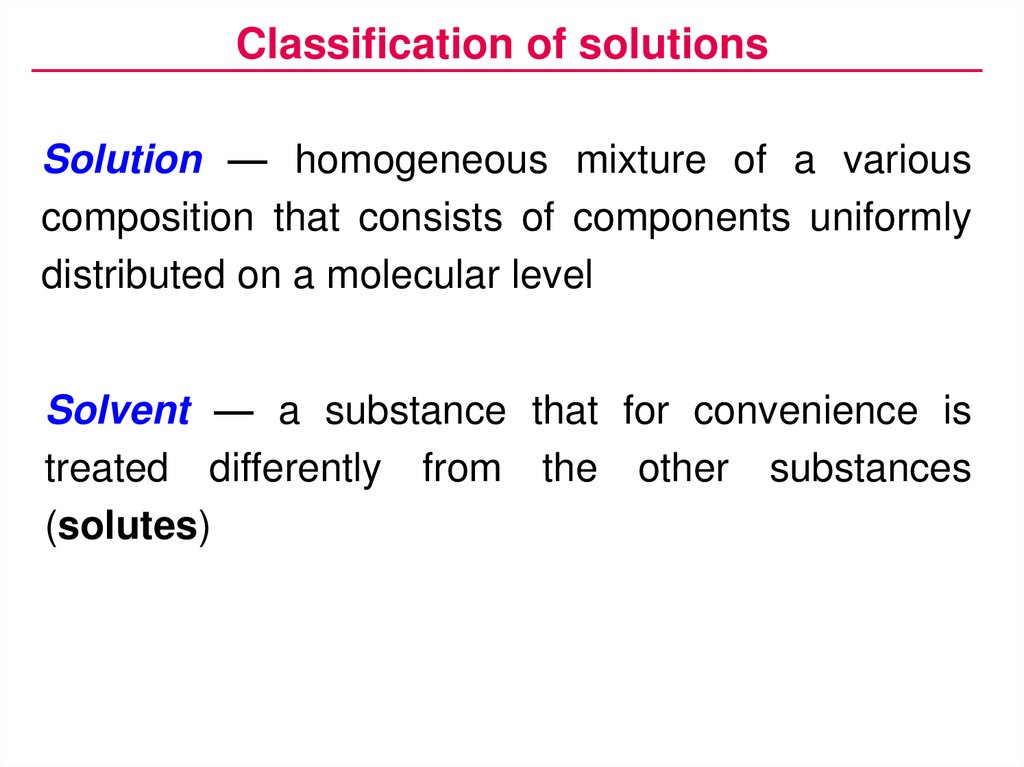# Solutions. Equilibrium in solutions of strong and weak electrolytes

## 1.

Lecture № 5
Solutions. Equilibrium in
solutions of strong and weak
electrolytes

## 2.

Context
1. Classification of solutions
2. Solutions and concentration
3. Strong and weak electrolytes
4. Colligative properties: osmosis
5. Osmosis
6. The pH concept
7. Strong acids and bases
8. Acids and bases: different approaches
9. Conjugate acids and bases
10. Weak acids and bases
11. pH in solutions of strong and weak acids
12. Solutions of weak acids, weak bases and salts

## 3.

Classification of solutions
Solution — homogeneous mixture of a various
composition that consists of components uniformly
distributed on a molecular level
Solvent — a substance that for convenience is
treated differently from the other substances
(solutes)

## 4.

Classification of solutions
Solution
Solid
Liquid
True
Gaseous
Colloid

## 5.

Classification of solutions
Solutions:
• aqueous and non-aqueous
• unsaturated , saturated, supersaturated
• diluted, concentrated
• solutions of electrolytes and non-electrolytes
• solutions of macromolecular compounds,
etc.

## 6.

Solutions and concentration
Mass fraction:
m(solute)
ω
( 100%)
m(solution)
Dimensionless (ω = 0.15) or percentage (ω = 15%)
Mole fraction:
Usually dimensionless
ni
χi
ni

## 7.

Solutions and concentration
Amount concentration
(molarity):
n(solute)
c
V (solution)
Mole per liter (mol/L or M);
Molality:
n(solute)
b
m(solvent)
Mole per kg;
for diluted solutions b[mol/kg] ≈ c[mol/L]

## 8.

Solutions and concentration
Example. Glucose (5.4 g) and sodium hydroxide
(0.6 g) were dissolved in water to give 100 ml of
solution with ρ = 1.05 g/ml. Calculate mass fractions,
mole fractions and amount concentrations of all
substances in the solution.
Name
Formula
Molar mass
Glucose
C6H12O6
180
Sodium
hydroxide
NaOH
40
Water
H2O
18
Mass
Amount

## 9.

Strong and weak electrolytes
Degree of dissociation (concentration dependent):
c diss
α
( 100%)
cinit
Strong electrolytes dissociate completely
( α > 30% in 0.1 M solution; usually α ≈ 100%):
NaCl → Na+ + Cl–
Weak electrolytes dissociate only to some extent
( α ≤ 30% in 0.1 M solution; usually α < 3%):
CH3COOH
CH3COO– + H+

## 10.

Strong and weak electrolytes
Strong electrolytes (α ≈ 100%)
Some acids:
Some bases:
HCl, HBr, HI, LiOH, NaOH, KOH,
HNO3, H2SO4,
RbOH, CsOH,
HClO4, HClO3 Ca(OH)2, Sr(OH)2,
Ba(OH)2
All salts:
NaCl, CuSO4,
Al2(SO4)3,
CH3COONH4,
etc.
Completely ionized in diluted solutions:
HCl → H+ + Cl–
H2SO4 → 2 H+ + SO42–
Ca(OH)2 → Ca2+ + 2 OH–
NaCl → Na+ + Cl–
CH3COONH4 → CH3COO– + NH4+

## 11.

Strong and weak electrolytes
Weak electrolytes (α < 3%)
Most acids:
Most bases:
HF, HCN, H2S, H2SO3,
H2CO3, HNO2, H3PO4, etc.,
all organic acids
NH3, amines, Cu(OH)2, etc.,
all amphoteric: Zn(OH)2,
Al(OH)3, Cr(OH)3, etc.
Reversible, stepwise ionization:
H + + F–
H+ + HSO3– (α1)
H+ + SO32– (α2)
α1 >> α2
NH3 + H2O
NH4+ + OH–
HF
(a) H2SO3
(b) HSO3–

## 12.

Colligative properties: osmosis
Semi-permeable membrane allows the solvent
molecules to pass through but holds back the solute
particles:
p
H2O
H2O
H2O
H2O
C6H12O6
H2O
C6H12O6
H2O
H2O
H2O
H2O
H2O

## 13.

Osmosis
Osmotic pressure — an excess pressure required
to maintain osmotic equilibrium between a solution
and the pure solvent separated by a membrane
permeable only to the solvent:
π = bosm RT ≈ cosm RT
Osmolarity (cosm) — the total molar concentration
of all solvated particles of the solutes in the
solution:
cosm = Σ(cn • in)

## 14.

Osmosis
Isotonic coefficient, or van't Hoff factor (i) — the
number of moles of particles (ions and/or
undissociated molecules) per mole of solute:
Solute type
Example
Non-electrolyte C6H12O6
Weak
electrolyte
Strong
electrolyte
CH3COOH
i
i=1
CH3COO– + H+
1<i<2
NaCl → Na+ + Cl–
i=2
CaCl2 → Ca2+ + 2 Cl–
i=3
Na3PO4 → 3 Na+ + PO43–
i=4

## 15.

Osmosis
Cell membrane and osmotic pressure:
c*ext
o
o
o
o
o
o
o
o
o
o
o
o
o o o o o o
o
o
o
o
oo oo o
o
o
o
o
o
o
o
o
o
o
o
o
oo
o
o
o
o
o
o
o
o
o
o
o
int
o
o
o
o
oo o o o o o
o
c*
o o
o
o o o o
o
o
o
o
o
o
o
o
o
o
o
o
o

## 16.

Osmosis
Plasmolysis and lysis (hemolysis) of erythrocytes:

## 17.

The pH concept
Water is a weak electrolyte:
aA + bB
K eq
H2O
H2O
cC + dD
[C]c [ D]d
[A]a [ B]b
H+ + OH–
H+ + OH–
ceq(X) = [X]
K
diss
[H ] [OH ]
[H 2O]
Kdiss (H2O)= 1.8 • 10–16 at 25 °C
1000 g
c(H2O) ≈ [H2O] = 18 g mol –1 = 55.6 mol/L
Kw = [H+] • [OH–] = 1.0 • 10–14 (at 25 °C)

## 18.

The pH concept
– lg[H+] – lg[OH–] = – lg(1.0 • 10–14) = 14
Kw = [H+] • [OH–] = 1.0 • 10–14 (at 25 °C)
pH = – lg [H+]
pOH = – lg [OH–]
pH + pOH = 14
in pure water pH = pOH = 7

## 19.

The pH concept
– lg[H+] – lg[OH–] = – lg(1.0 • 10–14) = 14
pH = – lg [H+]
pOH = – lg [OH–]
pH + pOH = 14
in pure water pH = pOH = 7

The pH concept
Strong acids:
Strong bases:
HX → H+ + X–
MOH → M+ + OH–
H2O
H+ + OH–
[H+] > [OH–]
pH < 7
pH = – lg
[H+]
H2O
H+ + OH–
[H+] < [OH–]
pH > 7
pOH = – lg [OH–]
pH = 14 – pOH

## 21.

Strong acids and bases
Example: The pH of 0.05 M solution of H2SO4
H2SO4 2 H+ + SO42–
pH = – lg [H+] = – lg
(2·0.05) = 1
Example: The pH of 0.01 M solution of NaOH
NaOH Na+ + OH–
pOH = – lg [OH–] = – lg
(0.01)= = 2
pH = 14 – pOH = 12

## 22.

Acids and bases: different approaches
The development of the acid-base theory
Concept
Acid
Base
Arrhenius
(1880)
Ionizes in water
to give H +
HCl
H+ + Cl–
Ionizes in water
to give OH –
NaOH
Na+ + OH–
Brønsted–Lowry
(1923)
A proton donor
HCl
H+ + Cl–
H 2O
H+ + OH–
A proton acceptor
NH 3 + H +
NH 4+
H2O + H+
H3O+
An electron pair
acceptor
An electron pair
donor
Lewis
(1930–1940)
H+,
AlCl 3 , etc.
OH– , H2O , NH3 , etc.

## 23.

Conjugate acids and bases
Conjugate acid-base pairs:
Conjugate acid ⇄ H+ + conjugate base
Conjugate base + H+ ⇄ conjugate acid
Water, the most important electrolyte:
conjugate
acid
H3O+
– H+
conjugate
base
H2O
– H+
+ H+ conjugate + H+
acid
OH–
conjugate
base
Example: Write conjugate acids and/or bases for the
following particles: CO32–, NH3, NH4+, HS–, H2S.

## 24.

Weak acids and bases
Acid-base equilibria in aqueous solutions
K
–logK
K w = [H + ] [OH – ] = 10 –14
14
[H+][A–]
Ka =
[HA]
pK a
[BH+][OH–]
Kb =
[B]
pK b
Equilibrium
H + + OH –
H2O
+
HA
H +A
B + H2O
BH
+

+
BH + OH
B+H
+
[H+] < C(HA)

K BH +
[B][H+]
=
[BH+]
[OH–] < C(B)
pK BH +

## 25.

pH in solutions of strong and weak acids
Strong acid:
HCl →
H2O
H+
+
Cl–
H+ + OH–
[H+] = c (HCl)
pH = – lg [H+]
= – lg c(HCl)
Weak acid:
HNO2
H2O
H+ + NO2–
H+ + OH–
[H+] < c (HNO2)
[H ] [A ]
Ka
[HA]
[H+] = [A–]; ca [HA]
[H ]2
Ka
ca
or pKa = 2pH + lgca
pH = ½[pKa – lg c(HNO2)]

## 26.

Solutions of weak acids, weak bases and salts
Electrolyte type (example)
Keq
Formula
pH
range
Weak acid
(CH3COOH)
Ka
Kw
pH = 1/2 (pKa – log c)
<7
Weak base
(NH3)
Kb
Kw
pH = 1/2 (14 + pKBH+ + log c)
>7
Salt of strong acid and strong base
(NaCl)
Kw
pH = pOH = 1/2 Kw = 7
=7
Salt of strong acid and weak base
(NH4Cl)
KBH+
Kw
pH = 1/2 (pKBH+ – log c)
<7
Salt of weak acid and strong base
(CH3COONa)
Ka
Kw
pH = 1/2 (14 + pKa + log c)
>7
Acidic salt of weak dibasic acid
(NaHCO3)
Ka1
Ka2
Kw
pH = 1/2 (pKa1 + pKa2)
~7
Amino acid
(NH2CH2COOH)